# Samacheer Kalvi Books: Tamilnadu State Board Text Books Solutions

## Samacheer Kalvi 12th Computer Science Chapter 2 Data Abstraction Notes PDF Download: Tamil Nadu STD 12th Computer Science Chapter 2 Data Abstraction NotesSamacheer Kalvi 12th Computer Science Chapter 2 Data Abstraction Notes PDF Download: Tamil Nadu STD 12th Computer Science Chapter 2 Data Abstraction Notes

Samacheer Kalvi 12th Computer Science Chapter 2 Data Abstraction Notes PDF Download: Students of class can download the Samacheer Kalvi 12th Computer Science Chapter 2 Data Abstraction Notes PDF Download from our website. We have uploaded the Samacheer Kalvi 12th Computer Science Chapter 2 Data Abstraction notes according to the latest chapters present in the syllabus. Download Samacheer Kalvi 12th Computer Science Chapter 2 Data Abstraction Chapter Wise Notes PDF from the links provided in this article.

## Samacheer Kalvi 12th Computer Science Chapter 2 Data Abstraction Notes PDF Download

We bring to you specially curated Samacheer Kalvi 12th Computer Science Chapter 2 Data Abstraction Notes PDF which have been prepared by our subject experts after carefully following the trend of the exam in the last few years. The notes will not only serve for revision purposes, but also will have several cuts and easy methods to go about a difficult problem.

 Board Tamilnadu Board Study Material Notes Class Samacheer Kalvi 12th Computer Science Subject 12th Computer Science Chapter Chapter 2 Data Abstraction Format PDF Provider Samacheer Kalvi Books

## How to Download Samacheer Kalvi 12th Computer Science Chapter 2 Data Abstraction Notes PDFs?

2. Click on the Samacheer Kalvi 12th Computer Science Notes PDF.
3. Look for your preferred subject.
4. Now download the Samacheer Kalvi 12th Computer Science Chapter 2 Data Abstraction notes PDF.

## Download Samacheer Kalvi 12th Computer Science Chapter 2 Data Abstraction Chapterwise Notes PDF

PART – I

Question 1.
Which of the following functions that build the abstract data type?
(a) Constructors
(b) Destructors
(c) Recursive
(d) Nested
(a) Constructors

Question 2.
Which of the following functions that retrieve information from the data type?
(a) Constructors
(b) Selectors
(c) Recursive
(d) Nested
(b) Selectors

Question 3.
The data structure which is a mutable ordered sequence of elements is called ………………………
(a) Built in
(b) List
(c) Tuple
(d) Derived data
(b) List

Question 4.
A sequence of immutable objects is called ………………………
(a) Built in
(b) List
(c) Tuple
(d) Derived data
(c) Tuple

Question 5.
The data type whose representation is known are called ………………………
(a) Built in data type
(b) Derived data type
(c) Concrete data type
(d) Abstract data type
(c) Concrete data type

Question 6.
The data type whose representation is unknown are called ………………………
(a) Built in data type
(b) Derived data type
(c) Concrete data type
(d) Abstract datatype
(d) Abstract datatype

Question 7.
Which of the following is a compound structure?
(a) Pair
(b) Triplet
(c) Single
(a) Pair

Question 8.
Bundling two values together into one can be considered as ………………………
(a) Pair
(b) Triplet
(c) Single
(a) Pair

Question 9.
Which of the following allow to name the various parts of a multi – item object?
(a) Tuples
(b) Lists
(c) Classes
(c) Classes

Question 10.
Which of the following is constructed by placing expressions within square brackets?
(a) Tuples
(b) Lists
(c) Classes
(b) Lists

PART – II

Question 1.
What is abstract data type?
Abstract Data type (ADT) is a type (or class) for objects whose behavior is defined by a set of values and a set of operations.

Question 2.
Differentiate constructors and selectors?
Constructors are functions that build the abstract data type. Selectors are functions that retrieve information from the data type.
To create a city object, you’d use a function like
city = makecity (name, lat, Ion)
To extract the information of a city object, you would use functions like
getname (city)

Question 3.
What is a Pair? Give an example?
Pair is a compound structure which is made up of list or Tuple.
lst[(0, 10), (1, 20)] -where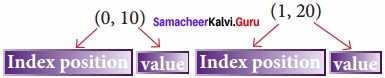Any way of bundling two values together into one can be considered as a pair. Lists are a common method to do so. Therefore List can be called a Pairs.

Question 4.
What is a List? Give an example?

• The list is constructed by placing expressions within square brackets separated by commas.
• Example: 1st := [10,20]

Question 5.
What is a Tuple? Give an example?
A tuple is a comma-separated sequence of values surrounded by parentheses. A tuple is similar to a list. The difference between the two is that you cannot change the elements of a tuple once it is assigned whereas, in a list, elements can be changed.
Example colour = (‘red’, ‘blue’, ‘Green’)

PART – III

Question 1.
Differentiate Concrete data type and abstract datatype?
Concrete data type:

1. A concrete data type is a data type whose representation is known.
2. Concrete data types or structures (CDT’s) are direct implementations of a relatively simple concept.

Abstract data type:

1. Abstract data type the representation of a data type is unknown.
2. Abstract Data Types (ADT’s) offer a high-level view (and use) of a concept independent of its implementation.

Question 2.
Which strategy is used for program designing? Define that Strategy?

• A powerful strategy for designing programs: ‘wishful thinking’.
• Wishful Thinking is the formation of beliefs and making decisions according to what might be pleasing to imagine instead of by appealing to reality.

Question 3.
Identify Which of the following are constructors and selectors?

• N1 = number ( ) – constructors
• Accetnum (n1) – selectors
• Displaynum (n1) – selectors
• eval (a/b) – selectors
• x, y = makeslope(m), makeslope (n) – constructors
• display() – selectors

Question 4.
What are the different ways to access the elements of a list? Give example?

• The elements of a list can be accessed in two ways.
• The first way is via our familiar method of multiple assignments, which unpacks a list into its elements and binds each element to a different name.
1st := [10, 20] x, y := 1st
In the above example, ‘x’ becomes 10 and ‘y’ becomes 20.
• A second method for accessing the elements in a list is by the element selection operator, also expressed using square brackets.
• Unlike a list literal, a square-brackets expression directly following another expression does not evaluate to a list value but instead selects an element from the value of the preceding expression.
• Example:
Ist
10
1st
20

Question 5.
Identify Which of the following are List, Tuple and class?
(a) arr [1, 2, 34]
(b) arr (1, 2, 34)
(c) student [rno, name, mark]
(d) day = (‘sun’, ‘mon’, ‘tue’, ‘wed’)
(e) x= [2, 5, 6.5, [5,6], 8.2]
(f) employee [eno, ename, esal, eaddress]
List: (a) arr [1, 2, 34]
(e) x= [2, 5, 6.5, [5,6], 8.2]
Tuple: (b) arr (1, 2, 34)
(d) day = (‘sun’, ‘mon’, ‘tue’, ‘wed’)
Class: (c) student [mo, name, mark]
(f) employee [eno, ename, esal, eaddress]

PART – IV

Question 1.
How will you facilitate data abstraction? Explain it with a suitable example?
The definition of ADT only mentions what operations are to be performed but not how these operations will be implemented. It does not specify how data will be organized in memory and what algorithms will be used for implementing the operations. It is called “abstract” because it gives an implementation-independent view. The process of providing only the essentials and hiding the details is known as abstraction.
To facilitate data abstraction, you will need to create two types of functions.

constructors and selectors:
Constructors are functions that build the abstract data type. Selectors are functions that retrieve information from the data type.
To create a city object, you’d use a function like city = makecity (name, lat, Ion)
To extract the information of a city object, you would use functions like

1. getname(city)
2. getlat(city)
3. getlon(city)

In the above pseudo code the function which creates the object of the city is the constructor, city = makecity (name, lat, Ion)
Here makecity (name, lat, Ion) is the constructor which creates the object city.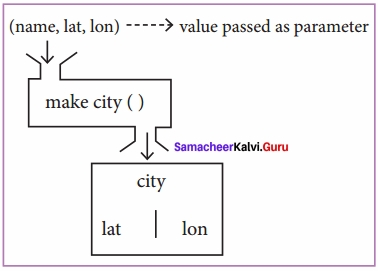Selectors are nothing but the functions that retrieve information from the data type. Therefore in the above code

1. getname(city)
2. getlat(city)
3. getlon(city)

are the selectors because these functions extract the information of the city object.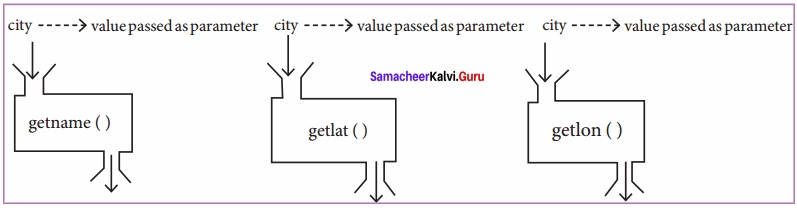Data abstraction is supported by defining an abstract data type (ADT), which is a collection of constructors and selectors. Constructors create an object, bundling together different pieces of information, while selectors extract individual pieces of information from the object.

Question 2.
What is a List? Why List can be called as Pairs. Explain with suitable example?
List is constructed by placing expressions within square brackets separated by commas. Example for List is [10, 20],
The elements of a list can be accessed in two ways. The first way is via our familiar method of multiple assignment, which unpacks a list into its elements and binds each element to a different name.
1st: = [10, 20]
x, y: = 1st
In the above example x will become 10 and y will become 20.
A second method for accessing the elements in a list is by the element selection operator, also expressed using square brackets. Unlike a list literal, a square – brackets expression directly following another expression does not evaluate to a list value, but instead selects an element from the value of the preceding expression.
1st 
10
1st 
20
In both the example mentioned above mathematically we can represent list similar to a set.
1st [(0, 10), (1, 20)] – where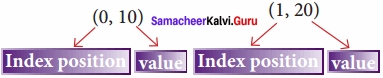Any way of bundling two values together into one can be considered as a pair. Lists are a common method to do so. Therefore List can be called as Pairs.

Question 3.
How will you access the multi-item? Explain with example?
List allows data abstraction in that you can give a name to a set of memory cells. For instance, in the game Mastermind, you must keep track of a list of four colors that the player guesses. Instead of using four separate variables (color 1, color2, color3, and color), you can use a single variable ‘Predict’, e.g.,
Predict = [‘red’, ‘blue’, ‘green’, ’green’]
What lists do not allow us to do is name the various parts of a multi-item object. In the case of a Predict, you don’t really need to name the parts:
using an index to get to each color suffices.
But in the case of something more complex, like a person, we have a multi-item object where each ‘item’ is a named thing: the firstName, the last Name, the id, and the email. One could use a list to represent a person.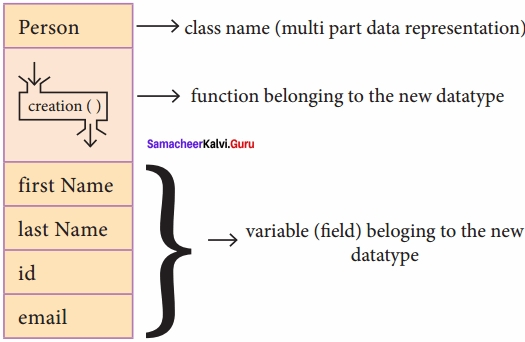but such a representation doesn’t explicitly specify what each part represents.
For this problem instead of using a list, you can use the structure constmct (In OOP languages it’s called class construct) to represent multi-part objects where each part is named (given a name). Consider the following pseudo code:
class Person:
creation( )
firstName: = “”
lastName: = ” ”
id: = ” ”
email : = “”
The new data type Person is pictorially represented as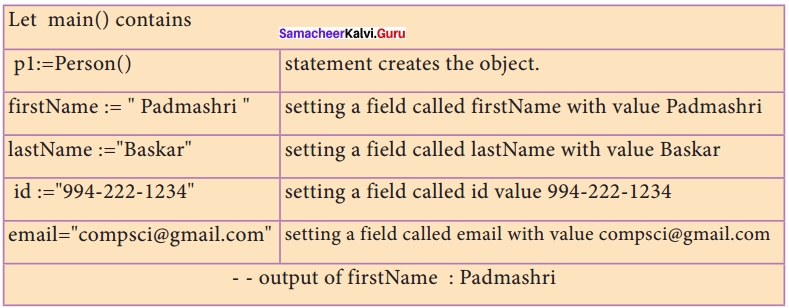The class (structure) constmct defines the form for multi – part objects that represent a person. Its defintion adds a new data type, in this case, a type named Person. Once defined, we can create new variables (instances) of the type. In this example, a Person is referred to as a class or a type, while p1 is referred to as an object or an instance. You can think of class Person as a cookie-cutter, and p1 as a particular cookie. Using the cookie cutter you can make many cookies. In the same way, using the class you can create many objects of that type.

### Samacheer Kalvi 12th Computer Science Data Abstraction Additional Questions and Answers

PART – 1

Question 1.
What allows data abstraction is that we can give a name to a set of memory cells.
a) Tuple
b) Set
c) Dictionary
d) List
d) List

Question 2.
(b) Application Developing Tool
(c) Abstract data types
(c) Abstract data types

Question 3.
The Splitting of the program into many modules are called as ……………………………
(a) Modularity
(b) Structures
(c) Classes
(d) List
(a) Modularity

Question 4.
The list can also be called as
a) Functions
b) Class
c) Pairs
d) Structure
c) Pairs

Question 5.
A linked list is of …………………………..
(a) Single
(b) Double
(c) Multiple
(d) Both a and b
(d) Both a and b

Question 6.
The process of providing only the essentials and hiding the details is known as …………………………..
(a) Modularity
(b) Structure
(c) Tuple
(d) Abstraction
(d) Abstraction

Question 7.
A powerful strategy for designing programs is ……………
a) Data Abstraction
b) ‘wishful thinking’
c) Concrete data type
d) Abstract Data Template
b)’wishful thinking’

Question 8.
: = is called as …………………………..
(a) Assigned as
(b) Becomes
(c) Both a and b
(d) None of these
(c) Both a and b

Question 9.
In list 1st [(0, 10), (1, 20)] – 0 and 1 represents …………………………..
(a) Value
(b) Index
(c) List identifier
(d) Tuple
(b) Index

Question 10.
Which of the following function that facilitates the data abstraction?
a) Constructors
b) Destructors
c) Selectors
d) a and c
d) a and c

Question 11.
nums  represent that you are accessing ………………………….. element.
(a) 0
(b) 1
(c) 2
(d) 3
(b) 1

Question 12.
nums  indicate that we are accessing ………………………….. element.
(a) 0
(b) 1
(c) 2
(d) many
(c) 2

Question 13.
Which provides modularity?
a) Data types
b) Subroutines
c) Abstraction
d) Classes
c) Abstraction

PART – II

Question 1.
Write a note on Data Abstraction

• Data abstraction is supported by defining an abstract data type (ADT) which is a collection of Constructors and Selectors.
• Constructors create an object, bundling together different pieces of information, while selectors extract individual pieces of information from the object.

Question 2.
Give the pseudo code to represent a rational number as a pair of two integers?
You can now represent a rational number as a pair of two integers in pseudo-code: a numerator and a denominator.
rational (n, d):
return [n, d]
numer (x):
return x 
denom (x):
return x 

Question 3.
What are the two ways of representing the pair data type?
Two ways of representing the pair data type. The first way is using the List construct and the second way to implement pairs is with the tuple construct.

Question 4.
Differentiate tuple and list?
List:
In List, the square bracket is used.

Tuple:
In Tuple, parenthesis is used.

Question 5.
Give an example for the representation of Tuple as a pair?
Representation of Tuple as a Pair
nums : = (1, 2)
nums 
1
nums 
2

Question 6.
Define class?
A class as bundled data and the functions that work on that data.

PART – III

Question 1.
Give the pseudo code to compute the distance between two city objects?
The following pseudo code will compute the distance between two city objects:
distance(city 1, city2):
1t1, 1g1: = getlat (city1), getlon (city1)
1t2, 1g2: = getlat (city2), getlon (city2)
return ((1t1 – 1t2) ** 2 + (1g1 – 1g2) ** 2)1/2

## How to Prepare using Samacheer Kalvi 12th Computer Science Chapter 2 Data Abstraction Notes PDF?

Students must prepare for the upcoming exams from Samacheer Kalvi 12th Computer Science Chapter 2 Data Abstraction Notes PDF by following certain essential steps which are provided below.

• Use Samacheer Kalvi 12th Computer Science Chapter 2 Data Abstraction notes by paying attention to facts and ideas.
• Pay attention to the important topics
• Refer TN Board books as well as the books recommended.
• Correctly follow the notes to reduce the number of questions being answered in the exam incorrectly
• Highlight and explain the concepts in details.

## Frequently Asked Questions on Samacheer Kalvi 12th Computer Science Chapter 2 Data Abstraction Notes

#### How to use Samacheer Kalvi 12th Computer Science Chapter 2 Data Abstraction Notes for preparation??

Read TN Board thoroughly, make separate notes for points you forget, formulae, reactions, diagrams. Highlight important points in the book itself and make use of the space provided in the margin to jot down other important points on the same topic from different sources.

#### How to make notes for Samacheer Kalvi 12th Computer Science Chapter 2 Data Abstraction exam?

Read from hand-made notes prepared after understanding concepts, refrain from replicating from the textbook. Use highlighters for important points. Revise from these notes regularly and formulate your own tricks, shortcuts and mnemonics, mappings etc.
Share: